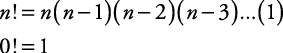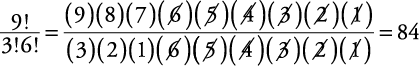## Factorials

Factorial is a convenient way of expressing the answer to any natural number multiplied together with all its preceding natural numbers. An exclamation point (!) is the symbol for a factorial. Thus “6!” is read as “six factorial.”

6! = (6)(5)(4)(3)(2)(1) = 720

Since zero is not a natural number, 0! would have no meaning. So that the expression 0! can be used to express formulas, it is defined as having the value of 1.##### Example 1

Evaluate.